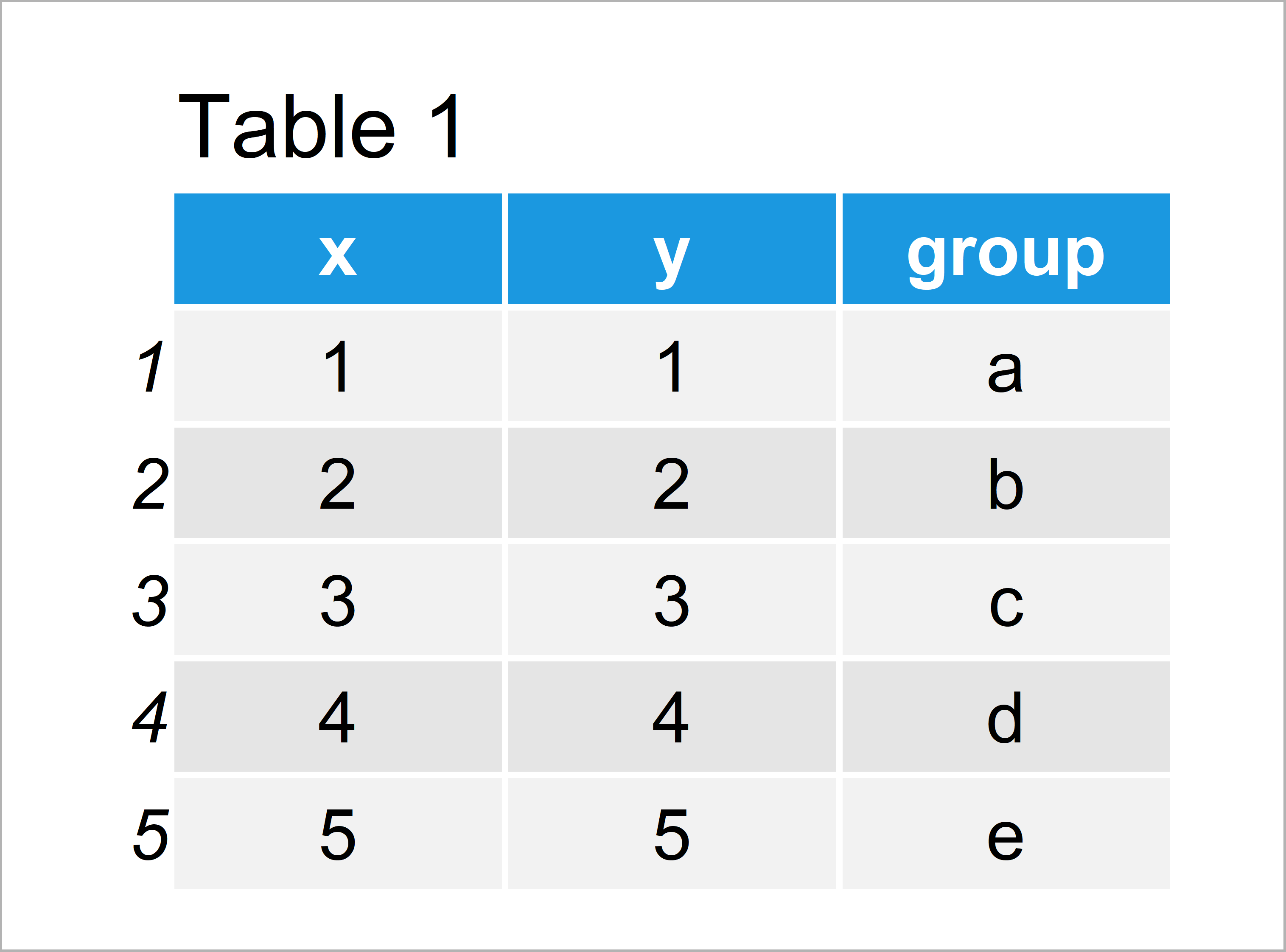# Draw ggplot2 Legend without Plot in R (Example)

In this tutorial, I’ll show how to create a ggplot2 legend without plot in R programming.

The article will contain these topics:

Here’s the step-by-step process:

## Example Data, Packages & Basic Graphic

First, we have to create some data that we can use in the examples later on:

```data <- data.frame(x = 1:5,                        # Create example data
y = 1:5,
group = letters[1:5])
data                                               # Print example data```Table 1 shows that our example data is constructed of five observations and three columns.

To be able to use the functions of the ggplot2 package, we also need to install and load ggplot2:

```install.packages("ggplot2")                        # Install ggplot2 package

Next, we can plot our data with a legend:

```ggp <- ggplot(data, aes(x, y, color = group)) +    # Create ggplot2 plot
geom_point(size = 5)
ggp                                                # Draw ggplot2 plot```In Figure 1 it is shown that we have plotted a regular ggplot2 scatterplot with a legend by executing the previous syntax.

## Example: Draw ggplot2 Legend without Plot Using grid, gridExtra & cowplot Packages

The following R programming code explains how to show only the legend of our plot without the actual plot itself.

For this, we first have to install and load three add-on packages to R: grid, gridExtra, and cowplot.

```install.packages("grid")                           # Install & load grid
library("grid")```
```install.packages("gridExtra")                      # Install gridExtra package
```install.packages("cowplot")                        # Install cowplot package

Next, we can apply the get_legend function of the cowplot package to extract the legend from our previously created ggplot2 plot:

`ggp_legend <- get_legend(ggp)                      # Save legend`

Finally, we can draw our legend without plot using the grid.newpage and grid.draw functions:

```grid.newpage()                                     # Draw empty plot window
grid.draw(ggp_legend)                              # Draw legend to window```## Video & Further Resources

Do you need further info on the R programming code of this article? Then you could watch the following video of the Statistics Globe YouTube channel. I illustrate the topics of this tutorial in the video.

Additionally, you may have a look at some of the related articles on my website. A selection of tutorials can be found below.